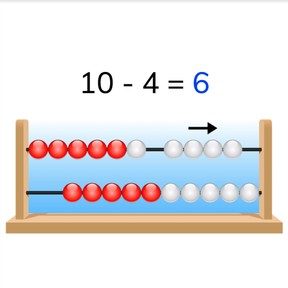Subtraction to 10 with rekenrek

# Subtraction to 10 with rekenrek

No account needed.8,000 schools use Gynzy92,000 teachers use Gynzy1,600,000 students use Gynzy

## General

Students learn to subtract numbers to 10 by solving the problems on the rekenrek.

1.OA.A

## Relevance

It is useful to be able to solve subtraction problems on the rekenrek so you can see how many beads are taken away and how many beads are left. Learning to use the rekenrek well also helps build mental math skills.

## Introduction

Students practice counting of quantities to 10. They count all the the similar objects in the image and jot down their totals. Discuss how many of each object there are and write the totals on the interactive whiteboard.

## Development

Check that students are able to subtract numbers to 10 on the rekenrek by asking the following questions:
- How can you quickly see how many beads are shown on the rekenrek?
- How many beads do you see on the top row of the rekenrek? And the bottom row? How many beads are there together?
- Which way do you move the beads if you take away one number from a second number?

## Guided practice

Students practice subtraction with numbers to 10 on the rekenrek. They must carefully look at the beads shown on the rekenrek in the images given.

## Closing

Have students work in pairs to practice subtraction on the rekenrek. Make sure that each pair has a rekenrek. The first student comes up with a subtraction problem for the rekenrek and says the subtraction problem aloud. The second student must solve the given problem. They take turns. Remind students that they must show leave space between the first number and the amount to take away, so you can clearly see the how much has been taken away. Practice together by asking students to match the subtraction problem to a rekenrek. Finally discuss that it is useful to be able to subtract with a rekenrek, so you build mental math skills.

## Teaching tips

Encourage students who have difficulty with subtraction with numbers to 10 to do all the subtraction problems from the lesson with you on the rekenrek. They can also be helped by working on recognizing amounts on the rekenrek. Then practice with simple subtraction in which only 1 is taken away each time (4 - 1, 3 - 1, etc). Some students may already be able to calculate mentally without using the rekenrek.

## Instruction materials

Rekenreks

### The online teaching platform for interactive whiteboards and displays in schools

• Save time building lessons

• Manage the classroom more efficiently

• Increase student engagement